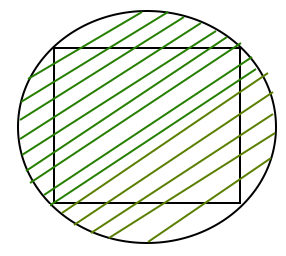# Area of a Circumscribed Circle of a Square

Given the side of a square then find the area of a Circumscribed circle around it.

Examples:

```Input : a = 6
Output : Area of a circumscribed circle is : 56.55

Input : a = 4
Output : Area of a circumscribed circle is : 25.13
```

## Recommended: Please try your approach on {IDE} first, before moving on to the solution.

All four sides of a square are of equal length and all four angles are 90 degree. The circle is circumscribed on a given square shown by a shaded region in the below diagram.Properties of Circumscribed circle are as follows:

• The center of the circumcircle is the point where the two diagonals of a square meet.
• Circumscribed circle of a square is made through the four vertices of a square.
• The radius of a circumcircle of a square is equal to the radius of a square.

Formula used to calculate the area of inscribed circle is:
(PI * a * a)/2
where, a is the side of a square in which a circle is circumscribed.

How does this formula work?
We know area of circle = PI*r*r.

We also know radius of circle = (square diagonal)/2
Length of diagonal = ?(2*a*a)
Area = PI*r*r = (PI*a*a)/2

## C++

 `// C++ Program to find the ` `// area of a circumscribed circle ` `#include ` `#define PI 3.14159265 ` ` `  `float` `areacircumscribed(``float` `a) ` `{ ` `    ``return` `(a * a * (PI / 2)); ` `} ` ` `  `// Driver code ` `int` `main() ` `{ ` `    ``float` `a = 6; ` `    ``printf``(``" Area of an circumscribed circle is : %.2f "``, ` `           ``areacircumscribed(a)); ` `    ``return` `0; ` `} `

## Java

 `// Java program to calculate ` `// area of a circumscribed circle-square ` `import` `java.io.*; ` `class` `Gfg { ` `    ``// Utility Function ` `    ``static` `float` `areacircumscribed(``float` `a) ` `    ``{ ` `        ``float` `PI = ``3``.14159265f; ` `        ``return` `(a * a * (PI / ``2``)); ` `    ``} ` ` `  `    ``// Driver Function ` `    ``public` `static` `void` `main(String arg[]) ` `    ``{ ` `        ``float` `a = ``6``; ` `        ``System.out.print(``"Area of an circumscribed"` `                         ``+ ``"circle is :"``); ` `        ``System.out.println(areacircumscribed(a)); ` `    ``} ` `} ` ` `  `// The code is contributed by Anant Agarwal. `

## Python3

 `# Python3 Program to find the ` `# area of a circumscribed circle ` `PI ``=` `3.14159265` ` `  `def` `areacircumscribed(a): ` ` `  `    ``return` `(a ``*` `a ``*` `(PI ``/` `2``))  ` ` `  `# Driver code ` `a ``=` `6` `print``(``" Area of an circumscribed circle is :"``, ` `        ``round``(areacircumscribed(a), ``2``)) ` `         `  `# This code is contributed by Smitha Dinesh Semwal `

## C#

 `// C# Program to find the ` `// area of a circumscribed circle ` `using` `System; ` ` `  `class` `GFG { ` `     `  `    ``public` `static` `double` `PI= 3.14159265 ; ` ` `  `    ``static` `float` `areacircumscribed(``float` `a) ` `    ``{ ` `        ``return` `(a * a * (``float``)(PI / 2)); ` `    ``} ` `     `  `    ``// Driver code ` `    ``public` `static` `void` `Main() ` `    ``{ ` `        ``float` `a = 6;  ` `         `  `        ``Console.Write(``" Area of an circumscribed"` `                            ``+ ``" circle is : {0}"``,  ` `             ``Math.Round(areacircumscribed(a), 2)); ` `    ``}  ` `} ` ` `  `// This code is contributed by  ` `// Smitha Dinesh Semwal `

## PHP

 ` `

Output :

``` Area of an circumscribed circle is : 56.55
```

Don’t stop now and take your learning to the next level. Learn all the important concepts of Data Structures and Algorithms with the help of the most trusted course: DSA Self Paced. Become industry ready at a student-friendly price.

My Personal Notes arrow_drop_upCheck out this Author's contributed articles.

If you like GeeksforGeeks and would like to contribute, you can also write an article using contribute.geeksforgeeks.org or mail your article to contribute@geeksforgeeks.org. See your article appearing on the GeeksforGeeks main page and help other Geeks.

Please Improve this article if you find anything incorrect by clicking on the "Improve Article" button below.

Improved By : Smitha Dinesh Semwal, vt_m

Article Tags :
Practice Tags :

Be the First to upvote.

Please write to us at contribute@geeksforgeeks.org to report any issue with the above content.